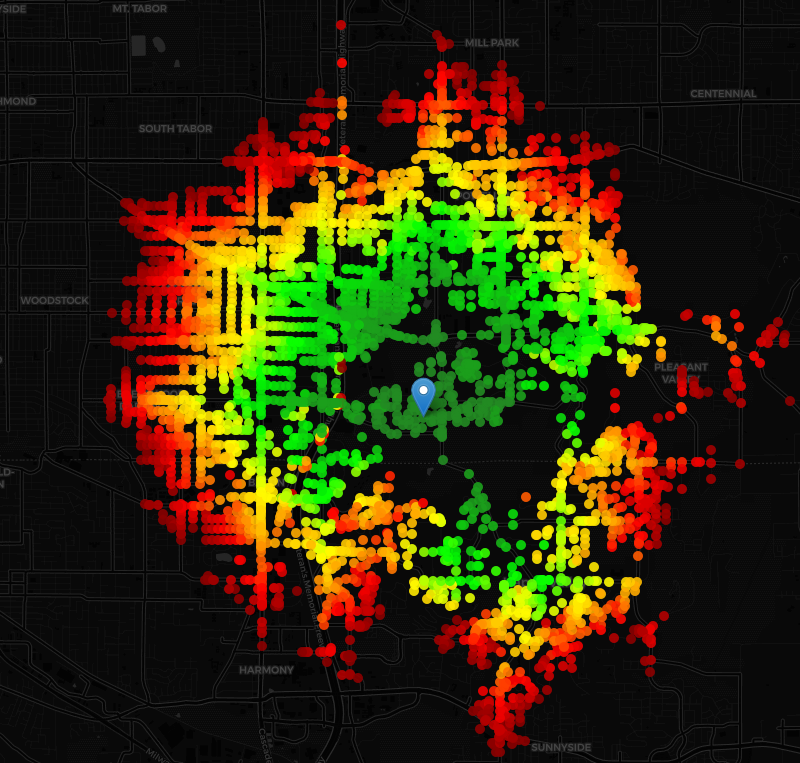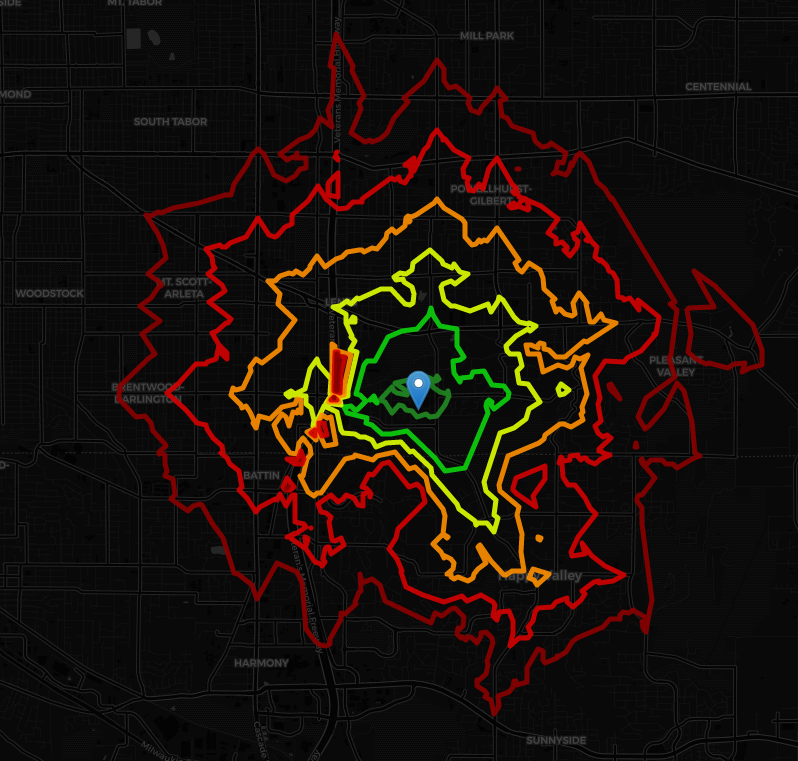# Fast contour lines using plain PostGIS

There’s a bunch of different ways to generate contour lines  from scatter data, each of them based on different interpolation algorithms. Like KNN , IDW  or kriging-flavoured methods . (image from Wikipedia)But, for performance sake, I’m going to use here a method derived from marching squares  method, that fits irregularly scattered data points: meandering triangles . The algorithm is pretty clearly described in the linked reference, so let’s go straight to the code.

First of all, our input is a dataset of points with a numeric value assigned to each of them. And, finally, a list of breaks for our data classification. You may want to hardcode the breaks to arbitrary values, or use some good ol’ data classification , but let’s keep it out of this function. So our input will be:

• geomin: geometries array
• colin: numeric values array
• breaks: numeric breaks array

And our output as a table of:

• geom: contour line geometry
• break: the break assigned to each contour line

First step, assign a bucket to each point using the input breaks, and store it in an array with the same sorting:

``````WITH
a as(
SELECT
width_bucket(t.x, breaks) as bin
FROM unnest(colin) as t(x)
)
SELECT array_agg(bin) INTO bucketin FROM a;
``````

Then, as the method’s name states, we need triangles instead of points! So let’s generate a TIN  from our scattered points using Delaunay Triangles , and store it in a geometries array.

``````WITH
a as (
SELECT unnest(geomin) AS e
),
b as (
SELECT ST_DelaunayTriangles(ST_Collect(a.e)) AS t FROM a
),
c as (
SELECT (ST_Dump(t)).geom AS v FROM b
)
SELECT array_agg(v) INTO gs FROM c;
``````

Now, let’s loop over the TIN and apply the meandering triangles algorithm to each cell. First of all, we need to identify the vertices of each cell, and the associated values from the original input arrays:

``````SELECT
array_agg(a.v),
array_agg(b.c),
array_agg(b.bk)
INTO
vertex, -- vertex geometry
vv, -- vertex value
bu -- vertex bucket
FROM
(
SELECT (ST_DumpPoints(g)).geom AS v
LIMIT 3 -- to drop the repeated 1st point
) as a
CROSS JOIN
LATERAL(
SELECT
t.*
FROM
unnest(geomin, colin, bucketin) as t(geo, c, bk)
WHERE ST_Equals(geo, a.v)
LIMIT 1
) as b;
``````

If the three vertices are in the same bucket, there’s no contour line crossing the cell:

``````CONTINUE WHEN bu = bu and bu = bu;
``````

If there are any intersection, let’s find them with our support function _get_cell_intersects (I will explain it later) that gets the intersections between the contour lines and the cell’s sides as a geometry array:

``````interp12 := _get_cell_intersects(vertex, vv, bu, breaks,1,2);
interp23 := _get_cell_intersects(vertex, vv, bu, breaks,2,3);
interp31 := _get_cell_intersects(vertex, vv, bu, breaks,3,1);
``````

Now that we have the points on each cell’s side that belongs to a contour line, let’s generate the segments that leave a different bucket value at each side of it. Take into account that there are 27 possible results (image from Wikipedia):So, taking into account the corner cases, the code to build the segments looks like:

``````WITH
a AS(
SELECT
t.*
FROM
unnest(breaks, interp12, interp23, interp31)
AS t(br, p12 , p23, p31)
),
b AS(
SELECT
CASE
WHEN
(p12 IS NOT NULL AND p23 IS NOT NULL AND ST_equals(p12, p23)=false) OR
(p23 IS NOT NULL AND p31 IS NOT NULL AND ST_equals(p23, p31)=false) OR
(p31 IS NOT NULL AND p12 IS NOT NULL AND ST_equals(p31, p12)=false)
THEN ST_MakeLine(ARRAY[p12, p23, p31]::geometry[])
ELSE null::geometry END AS  segm,
br
FROM a
)
SELECT
array_agg(b.segm) INTO segment
FROM unnest(breaks) AS c(x)
LEFT JOIN b ON b.br = c.x;
``````

Now that we have the segments, we add these segments to the calculated in previous iterations, collecting them per break value:

``````IF i = 0 THEN
running_merge = segment;
i := 1;
ELSE
WITH
a AS(
SELECT
ST_CollectionExtract(x, 2) AS x,
y
FROM unnest(running_merge,segment) AS t(x,y)
),
b AS(
SELECT
ST_collect(x,y) AS element
FROM a
)
SELECT
array_agg(element) INTO running_merge
FROM b;
END IF;
``````

Once we cycle through all the cells in our TIN, we need to sew our segments collections into proper lines and return them:

``````RETURN QUERY
WITH a AS(
SELECT
br,
ST_CollectionExtract(geo, 2) AS geo
FROM unnest(running_merge, breaks) AS t(geo, br)
),
b AS(
SELECT
ST_LineMerge(geo) AS geo,
br
FROM a
)
SELECT
geo AS geom,
br AS break
FROM b;
``````

So, the final function should look like:

``````CREATE OR REPLACE FUNCTION contour_lines(
IN geomin geometry[],
IN colin numeric[],
IN breaks numeric[]
)
RETURNS TABLE(geom geometry, break numeric)   AS \$\$
DECLARE
bucketin integer[];
gs geometry[];
g geometry;
vertex geometry[];
vv numeric[];
bu integer[];
inter numeric[];
interp12 geometry[];
interp23 geometry[];
interp31 geometry[];
segment geometry[];
running_merge geometry[];
i integer;
BEGIN
WITH
a AS(
SELECT
width_bucket(t.x, breaks) AS bin
FROM unnest(colin) AS t(x)
)
SELECT array_agg(bin) INTO bucketin FROM a;

WITH
a AS (SELECT unnest(geomin) AS e),
b AS (SELECT ST_DelaunayTriangles(ST_Collect(a.e)) AS t FROM a),
c AS (SELECT (ST_Dump(t)).geom AS v FROM b)
SELECT array_agg(v) INTO gs FROM c;

i:= 0;

FOREACH g IN ARRAY gs
LOOP

SELECT
array_agg(a.v),
array_agg(b.c),
array_agg(b.bk)
INTO vertex, vv, bu
FROM
(
SELECT (ST_DumpPoints(g)).geom AS v limit 3
) as a
CROSS JOIN
LATERAL(
SELECT
t.*
FROM
unnest(geomin, colin, bucketin) AS t(geo, c, bk)
WHERE ST_Equals(geo, a.v)
LIMIT 1
) AS b;

CONTINUE WHEN bu = bu and bu = bu;

interp12 := _get_cell_intersects(vertex, vv, bu, breaks,1,2);
interp23 := _get_cell_intersects(vertex, vv, bu, breaks,2,3);
interp31 := _get_cell_intersects(vertex, vv, bu, breaks,3,1);

WITH
a AS(
SELECT
t.*
FROM
unnest(breaks, interp12, interp23, interp31) AS t(br, p12 , p23, p31)
),
b AS(
SELECT
CASE
WHEN
(p12 IS NOT NULL AND p23 IS NOT NULL AND ST_equals(p12, p23)=false) OR
(p23 IS NOT NULL AND p31 IS NOT NULL AND ST_equals(p23, p31)=false) OR
(p31 IS NOT NULL AND p12 IS NOT NULL AND ST_equals(p31, p12)=false)
THEN ST_MakeLine(ARRAY[p12, p23, p31]::geometry[])
ELSE null::geometry END AS segm,
br
FROM a
)
SELECT
array_agg(b.segm) into segment
FROM unnest(breaks) AS c(x)
LEFT JOIN b ON b.br = c.x;

IF i = 0 THEN
running_merge = segment;
i := 1;
ELSE
WITH
a AS(
SELECT
ST_CollectionExtract(x, 2) AS x,
y
FROM unnest(running_merge,segment) AS t(x,y)
),
b AS(
SELECT
ST_collect(x,y) AS element
FROM a
)
SELECT
array_agg(element) INTO running_merge
FROM b;
END IF;

END LOOP;

RETURN QUERY
WITH a AS(
SELECT
br,
ST_CollectionExtract(geo, 2) AS geo
FROM unnest(running_merge, breaks) AS t(geo, br)
),
b AS(
SELECT
ST_LineMerge(geo) AS geo,
br
FROM a
)
SELECT
geo AS geom,
br AS break
FROM b;

END;
\$\$ LANGUAGE PLPGSQL IMMUTABLE;
``````

And the support function, that finds the intersections between the contour lines and a side of a cell, based on the cell data and the indexes of the vertices that defines that side:

``````CREATE OR REPLACE FUNCTION _get_cell_intersects(
IN vertex geometry[], -- vertices geometries
IN vv numeric[], -- vertices values
IN bu integer[], -- vertices buckets
IN breaks numeric[], -- breaks
IN i1 integer, -- first vertex index
IN i2 integer -- last vertex index
)
RETURNS geometry[]  AS \$\$
DECLARE
result geometry[];
BEGIN
-- init the array of results
result := array_fill(null::geometry, breaks::int[]);

-- if the vertices buckets are different,
-- there are might be intersections
IF bu[i1] <> bu[i2] THEN
SELECT
array_agg(b.point) INTO result
FROM
(
SELECT
-- linear interpolation of vertices values
-- to find the break point
(t.x-vv[i1])/(vv[i2]-vv[i1]) AS p
FROM unnest(breaks) AS t(x)
) AS a
LEFT JOIN LATERAL
(
SELECT
ST_LineInterpolatePoint(
ST_MakeLine(vertex[i1], vertex[i2]),
a.p
)
AS point
WHERE
-- we only want intersections between i1 and i2
a.p BETWEEN 0 AND 1
) AS b
ON 1=1;
END IF;

RETURN result;

END;
\$\$ LANGUAGE PLPGSQL IMMUTABLE;
``````

Updated 15-08-2019 to fix a typo in the support function, reported by @jacksonvoelkel. Once fixed, he sent a great use case via twitter. From thise raw data:To this contour lines:References:

Tags:

Categories:

Updated: